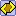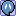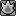#### quig101

i hate it when there are trick maps that look like the normal ones but aren't

#### gingbino11

i hate it when there are trick maps that look like the normal ones but aren't
The best one of them is when the is not not actually a lie, and everyone just runs at the door,

#### Aura Guardian

New features for the map editor.
<C><P G="0,-10" /><Z><S><S L="786" X="400" H="10" Y="31" T="2" P="0,0,0,1.2,0,0,0,0" /><S L="93" X="438" H="10" Y="384" T="0" P="0,0,0.3,0.2,0,0,0,0" /><S L="129" X="120" H="10" Y="188" T="1" P="0,0,0,0.2,0,0,0,0" /><S L="109" X="361" H="10" Y="123" T="1" P="0,0,0,0.2,0,0,0,0" /><S L="10" X="472" H="175" Y="187" T="1" P="0,0,0,0.2,0,0,0,0" /><S L="10" X="250" H="210" Y="194" T="1" P="0,0,0,0.2,0,0,0,0" /></S><D><F X="364" Y="162" /><T X="441" Y="380" /><DC X="643" Y="206" /><DS X="179" Y="322" /></D><O /></Z></C>

Also, how do we upload these maps from the text?

#### VKCA

##### (Virtual Circus Kareoky Act)
Why do you have to have 500 cheese to rate a map. That seems fucking stupid.
Doesn't affect me, but why does it matter how much cheese someone has?

#### Invective

have you seen how high of ratings some shit maps have?
and id assume its to keep the people who just started playing from voting for maps where they get cheese and against maps where the shaman actually has to do something (and only half of them get cheese).

#### zach the great

The players must have collected at least 500 cheeses to rate maps.
Good news.

#### Broth3r

Why do you have to have 500 cheese to rate a map. That seems fucking stupid.
Doesn't affect me, but why does it matter how much cheese someone has?
Mean only relatively experienced people get to vote, which hopefully will lead to lava/bounce/easy maps being voted out instead of getting 80%.

Although it should be 1000 cheese IMO.

#### internet

##### getting paid to moderateModerator
<C><P A="" /><Z><S><S X="267" H="20" Y="225" T="0" P="0,0,0.3,0.2,0,0,0,0" L="280" /><S X="521" H="20" Y="225" T="0" P="0,0,0.3,0.2,0,0,0,0" L="148" /><S X="404" H="20" Y="312" T="0" P="0,0,0.1,0.2,0,0,0,0" L="250" /><S X="531" H="87" Y="278" T="0" P="0,0,0.3,0.2,0,0,0,0" L="10" /></S><D><T Y="215" X="500" /><DS Y="200" X="269" /><F Y="269" X="423" /></D><O><O P="0" X="425" C="2" Y="268" /><O P="0" X="354" C="7" Y="288" /><O P="0" X="398" C="14" Y="293" /></O></Z></C>

#### Alchemator

##### my god if you don't have an iced tea for me when iOh webs that's pretty cool. Maybe slightly too difficult to get underneath, though I can still manage it pretty easily.

#### internet

##### getting paid to moderateModerator
Oh webs that's pretty cool. Maybe slightly too difficult to get underneath, though I can still manage it pretty easily.
thank you :3

#### SMZ

I finally could load the game (for some reason before I couldn't). ANYWAY I'm still fresh and stuff and my nickname is Metransform, cheesy I know.

#### Alchemator

##### my god if you don't have an iced tea for me when i<C><P /><Z><S><S L="726" X="421" H="73" Y="404" T="0" P="0,0,0.3,0.2,0,0,0,0" /></S><D><T X="85" Y="368" /><F X="679" Y="359" /><DS X="673" Y="130" /><DC X="544" Y="104" /></D><O><O P="0" X="552" C="2" Y="154" /><O P="0" X="745" C="2" Y="150" /><O P="0" X="746" C="2" Y="337" /><O P="90" X="747" C="4" Y="247" /><O P="0" X="650" C="4" Y="154" /><O P="0" X="747" C="11" Y="341" /><O P="0" X="746" C="11" Y="153" /><O P="0" X="554" C="11" Y="154" /><O P="70,0" X="560" C="4" Y="270" /><O P="0" X="527" C="14" Y="180" /><O P="0" X="83" C="2" Y="336" /><O P="0" X="85" C="10" Y="343" /><O P="0" X="85" C="22" Y="339" /><O P="0" X="198" C="2" Y="336" /><O P="0" X="303" C="2" Y="336" /><O P="0" X="199" C="10" Y="342" /><O P="0" X="304" C="10" Y="341" /><O P="0" X="304" C="22" Y="339" /><O P="0" X="199" C="22" Y="340" /></O></Z></C>

#### LeBron_James

This games awesome :D My names Lbjames

#### Quickster

So addicting. Username is Necroseer, pretty pro now. 5000+ cheese with nearly spidermouse (1000 firsts) :3

#### VKCA

##### (Virtual Circus Kareoky Act)
I thought 100 firsts was pirate mouse?

#### gingbino11

Man I have an awful first rate. ~3800 cheese and only 300 firsts.

Anyone got any tips to improve it?

#### reyscarfacepress ---> 3 seconds before the next map loads

#### Killah

also there have to be more than 11 mice in the room

and practice wall jumping- a lot

#### Xyphang

Tofuu, just got the hang of walljumping. I normally play /en because there's more people there.

And honestly, all the custom maps really suck. I find myself voting no on almost all of them.

#### Terminator

press ---> 3 seconds before the next map loads
The way the glitch works is that you have to get the cheese RIGHT AT 0.00, so when you start the next level, you'll have the cheese and can go directly into the hole before anyone else. You should do this on normal levels because if you do it on custom maps a) it's more difficult because of the time it takes to vote, and b) you start most official maps at the hole, so you will log out because of the 5 second rule.

#### reyscarfacebro im not talking about any glitches, i just told him to hold the arrow down so he can start running right away, since thats what most first-playing people do.

#### reyscarfacetHIS IS IMOPRTANT

WHY AM I LISTENING TO CAVE STORY AND THE CAN CAN WHEN PLAYING AAAAAAAAAAAAAA

#### zach the great

The above post is untrue.

#### Aura Guardian

Some of their mods can do music and have been able to for a long time.